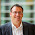## Saturday, February 9, 2013

### Finding a jump in data

The other day I saw a plot with some data with a clear jump in it. So I wondered if it were possible to determine the position of that jump. Data with a jump is easy enough made:
library(ggplot2)
n <- 100
mydata <- data.frame(x=runif(n))
mydata\$y <- rnorm(n,0,1) +ifelse(mydata\$x>.5,0,1)
qplot(x=mydata\$x,y=mydata\$y)

Now to find where that jump was. After some consideration, the most simple way seemed appropriate. Just take any possible point and examine the corrected sums of squares of the parts left and right of the break.
mydata <- mydata[order(mydata\$x),]
ss <- function(x) sum((x-mean(x))^2)
mids <- (mydata\$x[-1]+mydata\$x[-nrow(mydata)])/2
sa <- sapply(mids,function(x)
ss(mydata\$y[mydata\$x<x])+ss(mydata\$y[mydata\$x>x]))
qplot(x=mids,y=sa, ylab='Sum of squares',xlab='Position of break')
That was very simple. But still too complex. I realized I was recreating a tree with only one node. There is a way to get that first node more quickly:
library(tree)
tree(y~x,data=mydata,)

node), split, n, deviance, yval
* denotes terminal node

1) root 100 109.700  0.76210
2) x < 0.515681 58  50.430  1.23700
4) x < 0.0865511 7   8.534  0.56330 *
5) x > 0.0865511 51  38.280  1.33000
10) x < 0.144596 7   8.355  1.72600 *
11) x > 0.144596 44  28.650  1.26700
22) x < 0.276795 10   3.767  0.71330 *
23) x > 0.276795 34  20.920  1.43000
46) x < 0.380858 16  13.150  1.68100 *
47) x > 0.380858 18   5.856  1.20600 *
3) x > 0.515681 42  28.100  0.10580
6) x < 0.853806 29  13.950  0.28370 *
7) x > 0.853806 13  11.190 -0.29090
14) x < 0.938267 6   5.031 -0.70380 *
15) x > 0.938267 7   4.255  0.06312 *
So, the first break is at 0.51. That was easy.

#### In JAGS

I like JAGS far too much. So, I wanted to do that in JAGS. And it was simple too. Just make two means and (assume) a common standard deviation. The break (called limit when I programmed this, but break is an inconvenient name for a variable in R) has a beta prior.
library(R2jags)
model1 <- function() {
for ( i in 1:N ) {
category[i] <- (xx[i]>limit) + 1
yy[i] ~ dnorm( mu[category[i]] , tau )
}
tau <- 1/pow(sigma,2)
sigma ~ dunif(0,100)
mu ~ dnorm(0,1E-6)
mu ~ dnorm(0,1E-6)
limit ~ dbeta(1,1)
}
datain <- list(yy=mydata\$y,xx=mydata\$x,N=nrow(mydata))
params <- c('mu','sigma','limit')
inits <- function() {
list(mu = rnorm(2,0,1),
sigma = runif(1,0,1),
limit=runif(1,0,1))
}
jagsfit <- jags(datain, model=model1, inits=inits, parameters=params,
progress.bar="gui",n.iter=10000)
jagsfit

Inference for Bugs model at "C:/Users/.../Temp/RtmpuosigK/model176025bc6712.txt", fit using jags,
3 chains, each with 10000 iterations (first 5000 discarded), n.thin = 5
n.sims = 3000 iterations saved
mu.vect sd.vect    2.5%     25%     50%     75%   97.5%  Rhat n.eff
limit      0.528   0.042   0.474   0.504   0.516   0.541   0.629 1.001  3000
mu      1.212   0.128   0.959   1.128   1.213   1.296   1.465 1.002  1400
mu      0.122   0.152  -0.183   0.024   0.121   0.226   0.417 1.001  3000
sigma      0.922   0.069   0.795   0.872   0.917   0.967   1.070 1.002  1100
deviance 265.762   3.751 260.131 262.937 265.553 267.918 274.100 1.001  3000

For each parameter, n.eff is a crude measure of effective sample size,
and Rhat is the potential scale reduction factor (at convergence, Rhat=1).

DIC info (using the rule, pD = var(deviance)/2)
pD = 7.0 and DIC = 272.8
DIC is an estimate of expected predictive error (lower deviance is better).
So, the posterior median for the break is 0.516 with a slightly higher mean of 0.52. To make things more sweet, how about a nice combined plot? Extract the samples, take a hint from stackoverflow how to arrange the parts and a hint from google groups ggplot2 on the removal of the x=axis label. Mission accomplished.

limit = as.numeric(jagsfit\$BUGSoutput\$sims.array[,,'limit'])

q1 <- qplot(x=mydata\$x,y=mydata\$y,xlim=c(0,1),ylab='y') +
theme(axis.text.x = element_blank(),axis.title.x=element_blank(),
axis.ticks.x=element_blank())
d1 <- ggplot(,aes(x=limit)) + geom_density() +
scale_x_continuous(limits=c(0,1),'x') +
scale_y_continuous('Density of break position' )

library(gridExtra)
grid.arrange(q1,d1, nrow=2)

1.I like the use of tree regression a lot.

R also has some very nice implementations of broken stick regression, which will give you a break in a linear trend.

piecewise.linear()
bent.cable()

2.As above there are several packages devoted to the problem of detecting jumps in data. The one that springs to mind is strucchange for changes in regression. The advantage of this package is that you can enter many explanatory variables, just as you would to the lm function.

1.That sounds like the package I should have found. But I didn't. Thanks.